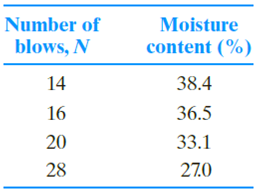Chapter 4, Problem 4.2PPrinciples of Geotechnical Enginee...

9th Edition
Braja M. Das + 1 other
ISBN: 9781305970939

Solutions

Chapter
SectionPrinciples of Geotechnical Enginee...

9th Edition
Braja M. Das + 1 other
ISBN: 9781305970939
Textbook Problem

Refer to the soil in Problem 4.1. A second group of students conducted only one test and found that the groove on the soil sample closed 12.5 mm when N = 21 and w = 30.4%. Estimate the liquid limit by the one-point method.4.1 During Atterberg limit tests in the soil mechanics laboratory, the students obtained the following results from a clayey soil.Liquid limit tests:Plastic limit tests: Students conducted two trials and found that PL = 17.2% for the first trial and PL = 17.8% for the second trial.a. Draw the flow curve and obtain the liquid limit.b. What is the plasticity index of the soil? Use an average value of PL from the two plastic limit trails.

To determine

Find the liquid limit value by one point method.

Explanation

Given information:

The groove on the soil sample, when the number of blows (N) is 21 and the moisture content (w) of 30.4 % is 12.5 mm.

Calculation:

Determine the liquid limit value by one point method.

LL=w(N25)tanβ

Substitute 30.4 % for w, 21 blows for N, and 0.121 for tanβ

Still sussing out bartleby?

Check out a sample textbook solution.

See a sample solution

The Solution to Your Study Problems

Bartleby provides explanations to thousands of textbook problems written by our experts, many with advanced degrees!

Get Started

What is a DBMS, and what are its functions?

Database Systems: Design, Implementation, & Management

What is an RFP, and how does it differ from an RFQ?

Systems Analysis and Design (Shelly Cashman Series) (MindTap Course List)

List types of productivity applications.

Enhanced Discovering Computers 2017 (Shelly Cashman Series) (MindTap Course List)

List some applications that use medium-pressure piping.

Welding: Principles and Applications (MindTap Course List)